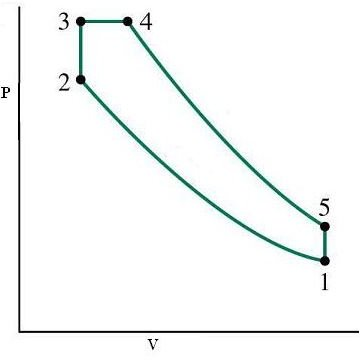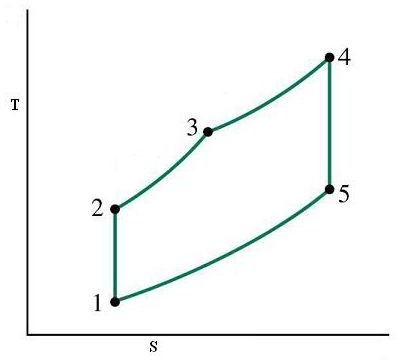# Theoretical Cycles in Marine Diesel Engines – The Dual Cycle

## Introduction

We studied about the Otto cycle and Diesel cycles in the previous articles and in this article we will move further by studying the dual cycle upon which is a combination of Otto and diesel cycles. As before, just remember that these are ideal cycles not fully followed by real engines and use air as the standard working substance.

## The Dual Cycle

As we saw in the previous two types of cycles namely the Otto cycle and the diesel cycle that they differ in the manner in which heat is added to the cycle either at constant volume or at constant pressure. In actual slow speed diesel engines (which as we shall see elsewhere in this channel are the ones used for the main propulsion plants of ships) have sufficient time period for this heat addition, hence the actual transfer of heat can be more accurately imitated by assuming part of the heat addition taking place at a constant volume and part at constant pressure thus giving the name of mixed or dual cycle to this type of cycle, and it combines the advantages of both these types of cycles.

As before just integrate this text with the accompanying diagrams of P-V and T-S and learn about this cycle.

• 1-2 is the isentropic compression which results in temperature rise, volume decrease and pressure rise of the working substance i.e. air
• 2-3 is the process where heat is added at a constant volume to the cycle just like in the Otto cycle
• 3-4 is the process where part of the heat added in the previous process is continued to be added but now at a constant pressure instead of a constant volume (thus the name dual mode) like in the case of diesel cycle
• 4-5 represents the isentropic expansion of air
• 5-1 is the heat rejection at constant volume thus bringing the full cycle to a completion and ready for the next cycle

Hence it can be seen that instead of 4 processes, we have 5 processes BUT in case you are jumping to a conclusion that it is a 5 stroke cycle you are wrong. It is still a four stroke cycle, the only difference being that the second stroke takes place in two different phases instead of one.

The following ratios are defined for this cycle for the purposes of calculating thermal efficiency

r the normal compression ratio = V1/V2

rc is the cut off ratio = V4/V3

rp is the pressure ratio = P3/P2

Thermal efficiency = 1 – {(T5 – T1)/(T3 – T2) + k(T4 – T3)}

OR

Thermal efficiency = 1 – (1/r)^(k-1) * {rp * rc^k – 1/(rp-1) + rp*k * (rc – 1)}

Where k is a constant depending on the properties of the working substance and T is the temperature at various points as shown in the diagrams below

We will compare the three cycles studied so far in the next article

## P-V and T-S Diagrams for a Dual Cycle